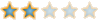Montreal Math ClubMathematics students in Montreal - Étudiants de mathématiques à MontréalHomeCalendarFAQSearchMemberlistUsergroupsRegisterLog in

 Montreal Math Club :: Chit chat :: The Water Cooler Share

# Division by ZeroAuthorMessage

EuclidPosts : 95
Join date : 2009-09-15
Age : 57
Location : AlexandriaSubject: Division by ZeroMon Sep 28, 2009 10:16 am A nice example of division by zero may be found at chapter 141. in Burnside's "Theory of Finite Groups".Let i be a primitive root of the congruence i^(p^m -1) = 1 (modulo p).Let a, b, c, d be powers of i with ad-bc <> 0 (modulo p).Consider the operations (ax+b)/(cx+d) (modulo p).Quote :Moreover, if we represent i^x/0 by infinity for all values of x, any operation of this group, when carried out on the set of quantities. infinity, 0, i, i^2,......, i^(p^m-1),will change each of them into another of the set; [...]Hence the permutation-group is triply transitive, since it contains an operation transforming any three of the p^m + 1 symbols into any other three.EuclidPosts : 95
Join date : 2009-09-15
Age : 57
Location : AlexandriaSubject: Re: Division by ZeroWed Nov 10, 2010 1:42 pm Another OneLast Sunday, one of our colleagues presented a nice division by zero in the very applied probabilities domain.Biasing the very clear presentation, that definitively deserves an accessible to students published note, I will say that was about the :dF/dGdivision where, par Newton!, dF and dG are infinitesimally small quantities.Par l'Hôspital! dF/dG makes sense and dF/dG = F'/G', even when F and G are probabilities.In practice, to apply l'Hôspital's rule to zero-probability events requires - A missing definition (that is equivalent to introducing infinitesimal dP probabilities) - A postulate that the definition fits to the real insurance world as well as the Topology and the Theory of Measure, and - A double column paper comparing the dF calculus to the delta-epsilon calculus.By the way, here is a less known construction of the real numbers,Definition: A real number is an equivalence class of slopes.where a slope is a map L : Z -> Z, with the property that the set {L(m + n) − L(m) − L(n) | m, n in Z} is finite.Division by ZeroPage 1 of 1

Permissions in this forum:You cannot reply to topics in this forumJump to: Select a forum||--Math Club|   |--Mathematical Sundays|   |--Suggestions|   |--Introduce yourself|   |--Mathematics|   |--Problem Solving|   |   |--Solved problems|   |   |   |--Calculus and Analysis|   |--Combinatorics|   |--Geometry|   |--Number Theory|   |--Probabilities and statistics|   |--Set theory and Topology|   |--Online Resources|   |--Chit chat    |--The Water Cooler    |--Mathematical poems